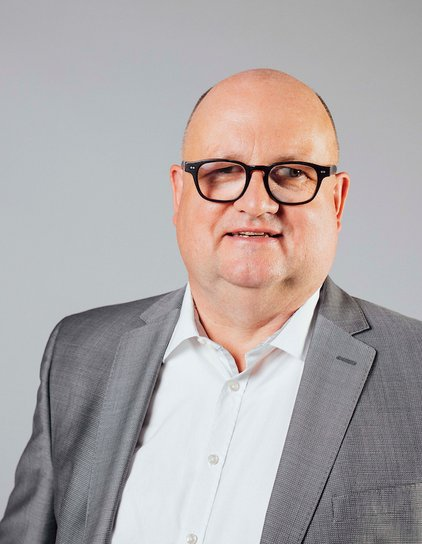# Group Leader of "Computational Methods in Systems and Control Theory"## Prof. Dr. Peter Benner

Main focus

• Numerical linear algebra
• Model reduction and system approximation
• Parallel algorithms and mathematical software
• Nonlinear equations, in particular algebraic Riccati equations